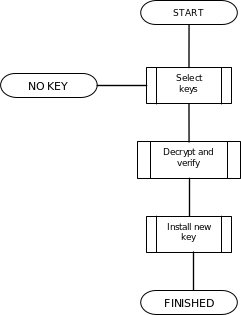## F.4 DU

31.1133GPPRelease 8TSUniversal Subscriber Identity Module Application Toolkit (USAT) interpreter byte codes

### F.4.1 Plug-in Execution

The flow diagram below illustrates briefly the different steps of the DU execution.Figure F.4: DU Flow Diagram

The termination states shall be mapped to output variables according to:

 State Plug-in Status Code Functional Output Description FINISHED "PS: OK" – Indicates success. NO KEY "PS: No such key" “error:noKey” The requested key was not available.

In case of a serious error not listed above, an implementation may use any of the Error Codes listed in the error code table in subclause 8.8.

Installing the new key means simply copying the key material to the location referenced by key index input parameter.

### F.4.2 Decryption and Verification Procedure

This procedure includes decryption of the encrypted key data, as well as verification of its authenticity.

To decrypt and verify the key data, select the correct algorithm based on the algorithm identifier field and thereafter proceed according to the selected algorithm.

An implementation shall support at least one algorithm.

Algorithms employing SHA-1 are preferred prior to algorithms employing ISO/IEC 9797.

#### F.4.2.1 3DES EDE CBC with two keys + SHA-1 MDC

The decrypted key data shall be formatted according to the table below.

 Bytes Description M/O Length 1 – 8 Random nonce. M 8 9 – P Key material M 16 or 24 Q – R Key checksum. M 8

The values P,Q and R are calculated from wrapped key length according to the following table:

 Wrapped key length P Q R 16 24 25 32 24 32 33 40

To decrypt and verify the key data, do the following:

1. Select the key pointed to by the key index input parameter. This is the destination key ,KD.
2. Based on the key index parameter, locate the unwrap key, KU.
3. Calculate the decrypted key data

DK = TDEA_DECR(Encrypted key data)

using the following cipher parameterisation:

 Keys K1 and K2 of KU. Cipher mode Outer CBC in EDE operation. IV ’00 … 00’ (this is not a weakness since the nonce effectively becomes a randomly chosen IV).
1. Calculate the message digest

MD = SHA1(unencrypted parameters || DK<1..P>)

The unencrypted parameters (‘Index of secret key’ and ‘Options’) shall be included in the checksum calculation to avoid certain replay attacks.

2. Calculate the key checksum

KC = MD<1..8>

3. Compare KC with DK<Q..R>. If identical, proceed to the next step. Otherwise, the plug-in shall set the Error Code to ‘Execution Error’ and terminate.
4. Success.

#### F.4.2.2 3DES EDE CBC with two keys + ISO/IEC 9797 MAC

The format of the decrypted key data is the same as in the previous subclause (F.4.2.1).

To decrypt and verify the key data, do the following:

1. Select the key pointed to by the key index input parameter. This is the destination key, KD.
2. Based on the key index parameter, locate the unwrap key, KU.
3. Calculate the decrypted key data

DK = TDEA_DECR(Encrypted key data)

using the following cipher parameterisation:

 Keys K1 and K2 of KU. Cipher mode Outer CBC in EDE operation. IV ’00 … 00’ (this is not a weakness since the nonce effectively becomes a randomly chosen IV).

PM = ISO_IEC_9797_PAD2(unencrypted parameters || DK<1..P>)

The unencrypted parameters (‘Index of secret key’ and ‘Options’) shall be included in the checksum calculation to avoid certain replay attacks.

2. Calculate the key checksum

KC = ISO_IEC_9797_ALG3(PM)

Using terminology from , keys K and K’ shall be derived by complementing alternate sub-strings of four bits of K1 and K2 respectively, commencing with the four most significant bits.

8 bytes of output from the MAC calculation shall be used (i.e. m=64 using ISO/IEC 9797 terminology).

3. Compare KC with DK<Q..R>. If identical, proceed to the next step. Otherwise, the plug-in shall set the Error Code to "Execution Error" and terminate.
4. Success.

#### F.4.2.3 3DES EDE CBC with three keys + SHA-1 MDC

This algorithm is identical to the algorithm described in F.4.2.1, except that the 3DES cipher shall be parameterized with three DES keys.

#### F.4.2.4 3DES EDE CBC with three keys + ISO/IEC 9797 MAC

This algorithm is identical to the algorithm described in F.4.2.2, except that the 3DES cipher shall be parameterized with three DES keys. For the MAC calculation, only K1 and K2 shall be used.